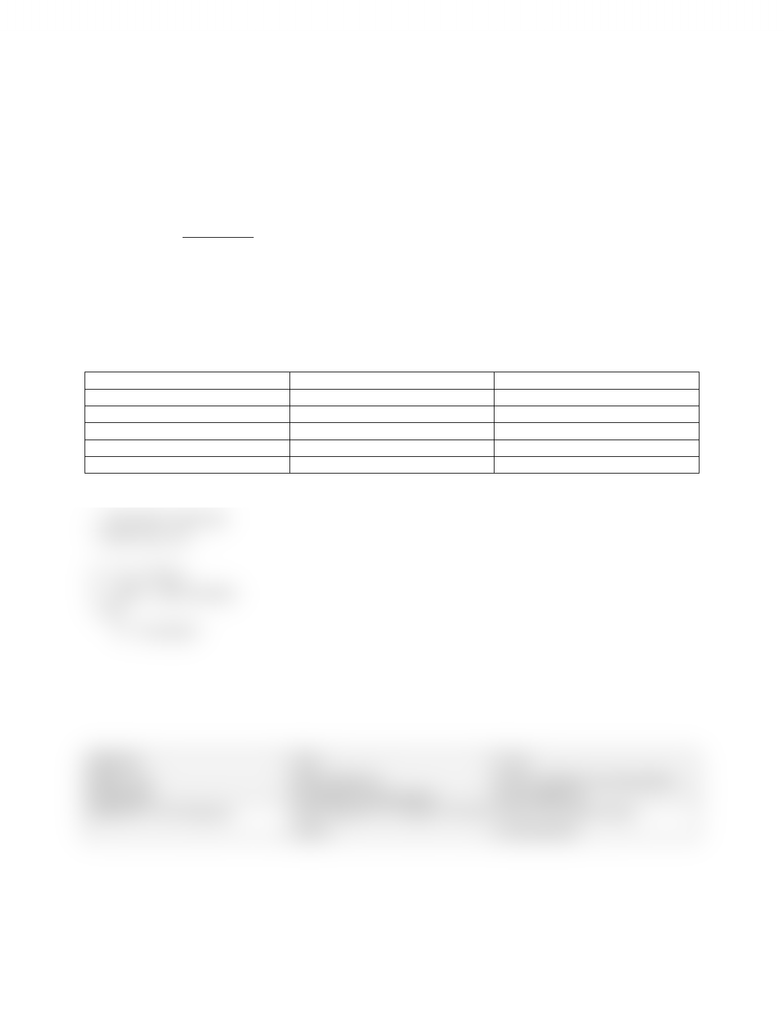# ACC 406 Lecture Notes - Scatter Plot, Fixed Cost, Variable Cost

40 views2 pagesChapter 3: Cost Behaviour
The relationship between cost and activity volume
Variable cost + fixed cost = total cost
3 methods of calculation:
High-low method
Scatterplot method
Method of least squares
To estimate the cost formula
Total Cost = Total Fixed Cost + (variable cost per unit) (activity unit)
Y = F+ (V) (X)
1) High Low Method
Month
Total Cost
Unit Produced
Jan (low)
\$2,000
200
Feb
\$2,500
400
March
\$4,500
600
April
\$5,000
800
May (high)
\$7,500
1000
Variable Method = YH-Y.L / XH-XL
= 7500-2000/ 1000-200
= \$6.875 per unit
F = YH- (V)(XH)
F = 2000 (\$6.875)(200)
= 625
2) Scatterplot
Methods
Pros
Cons
High- Low
More objective
Only considers two data points
Scatterplot
Considers all data points
Too subjective
Method of Least Squares
More objective, considers all data
points
Does not exclude others
automatically
Unlock document

This preview shows half of the first page of the document.
Unlock all 2 pages and 3 million more documents.